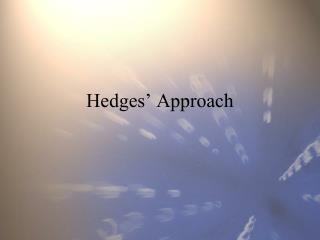DownloadDownload PresentationHedges’ Approach

# Hedges’ Approach

Download Presentation## Hedges’ Approach

- - - - - - - - - - - - - - - - - - - - - - - - - - - E N D - - - - - - - - - - - - - - - - - - - - - - - - - - -
##### Presentation Transcript

1. Hedges’ Approach

2. Two main camps in MA • Schmidt & Hunter • Hedges et al. • Hedges & Olkin • Hedges & Vevea • Differ in Weights and Data Transformation • Others – HLM, Rosenthal, Bayesian, not as common

3. Weights Defined • SH use N, NA2 for weights • Hedges uses inverse variance weights. • Sampling variances and inverses:

4. InV Weights Pros & Cons

5. Data Transformation r .10 .20 .30 .40 .50 .60 .70 .80 .90 z .10 .20 .31 .42 .55 .69 .87 1.10 1.47

6. Confidence Interval Because w=N-3, this basically means that the confidence interval is the mean plus or minus 2 times the root of 1/(Total N).

7. Homogeneity Test When the null (homogeneous rho) is true, Q is distributed as chi-square with (k-1) df, where k is the number of studies. This is a test of whether Random Effects Variance Component is zero.

8. Estimating the REVC If REVC estimate is less than zero, set to zero. REVC is SH Var(rho), but in the metric of z, not r. Method due to DerSimonian & Laird

9. Random-Effects Weights Inverse variance weights give weight to each study depending on the uncertainty for the true value of that study. For fixed-effects, there is only sampling error. For random-effects, there is also uncertainty about where in the distribution the study came from, so 2 sources of error. The InV weight is, therefore:

10. Numerical Illustration (1)

11. Numerical Illustration (2)

12. Numerical Illustration (3) Fixed-effects mean and CI: Retranslate to r: But, generally best to use RE, even if Q is n.s.

13. Numerical Illustration (4)

14. Numerical Illustration (5) Comparison of Results